# Lesson 20 Objects in JavaScript

So in the last few lessons, we have been talking about arrays which are a part of the data structure. In this lesson, we will discuss Objects in JavaScript which is also a part of data structure and we will also discuss what objects are and how we can use objects in our program.

## Objects in JavaScript

### Why we use Objects:

So let suppose that I wanted to store data of some person like its username, password, and its email address.
So these data are not related to each other they are totally different elements, so if I wanted to connect them I can use an array to store all these data like below code:

``````var person = ["Denis", "denis123", "denis@xyz.com"];
``````

So this not great way to store data because this data is not really a list.
This list is not in a logical order.
Like we want to access email address then I need to write person [index], and that requires me to know the index of every element that I want to retrieve.
So I am giving you this example to show you that array is not the perfect solution for every situation, In this situation, we use objects which is also a part of the data structure.
So you can see in the below example where we creating an object with variable name person which contain the name, username, email ID.
So here is the code below:

``````Var person = {username: “denis”,
Email ID "denis@xyz.com"};
``````

So you can see here we have three different items in this object, and there is built-in order, so just like arrays here we don’t need to care about index number, so here no property comes first or second, it does not matter how I declared them and in what order, they are all treated the same.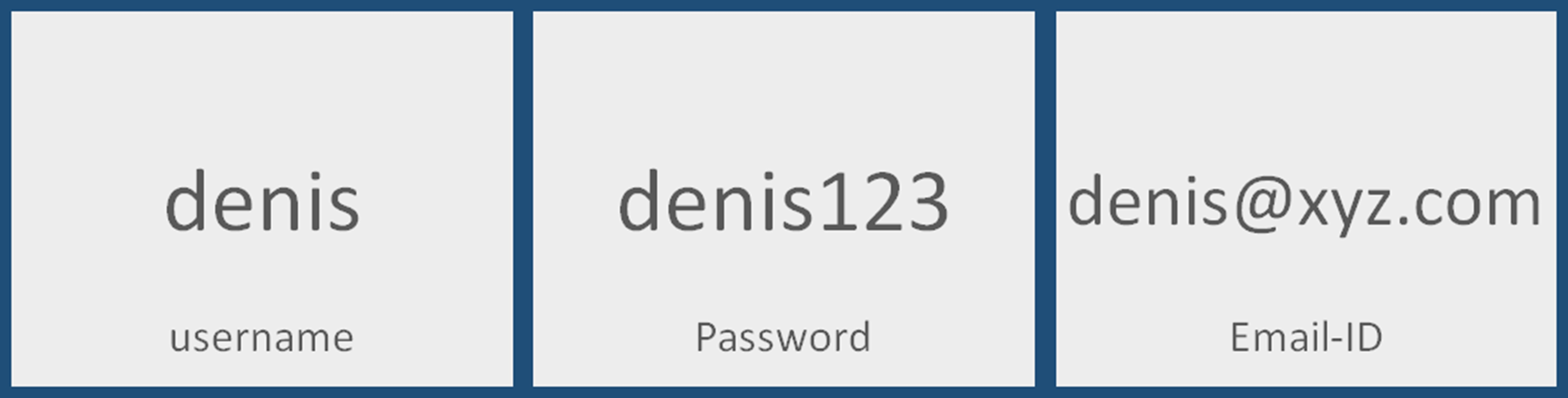So you can see “denis” is stored under the key username, denis123 stored under password and denis@xyz.com stored under Email-id
So we have two choices to retrieve data from outside of an object.
So we can either use the name of the object that is person in our case, so person[“username”].
Here below is the code to retrieve data.

``````var person = {
emailid: "denis@xyz.com"
};
//to retrieve name we use
``````

Here is the output below of above code: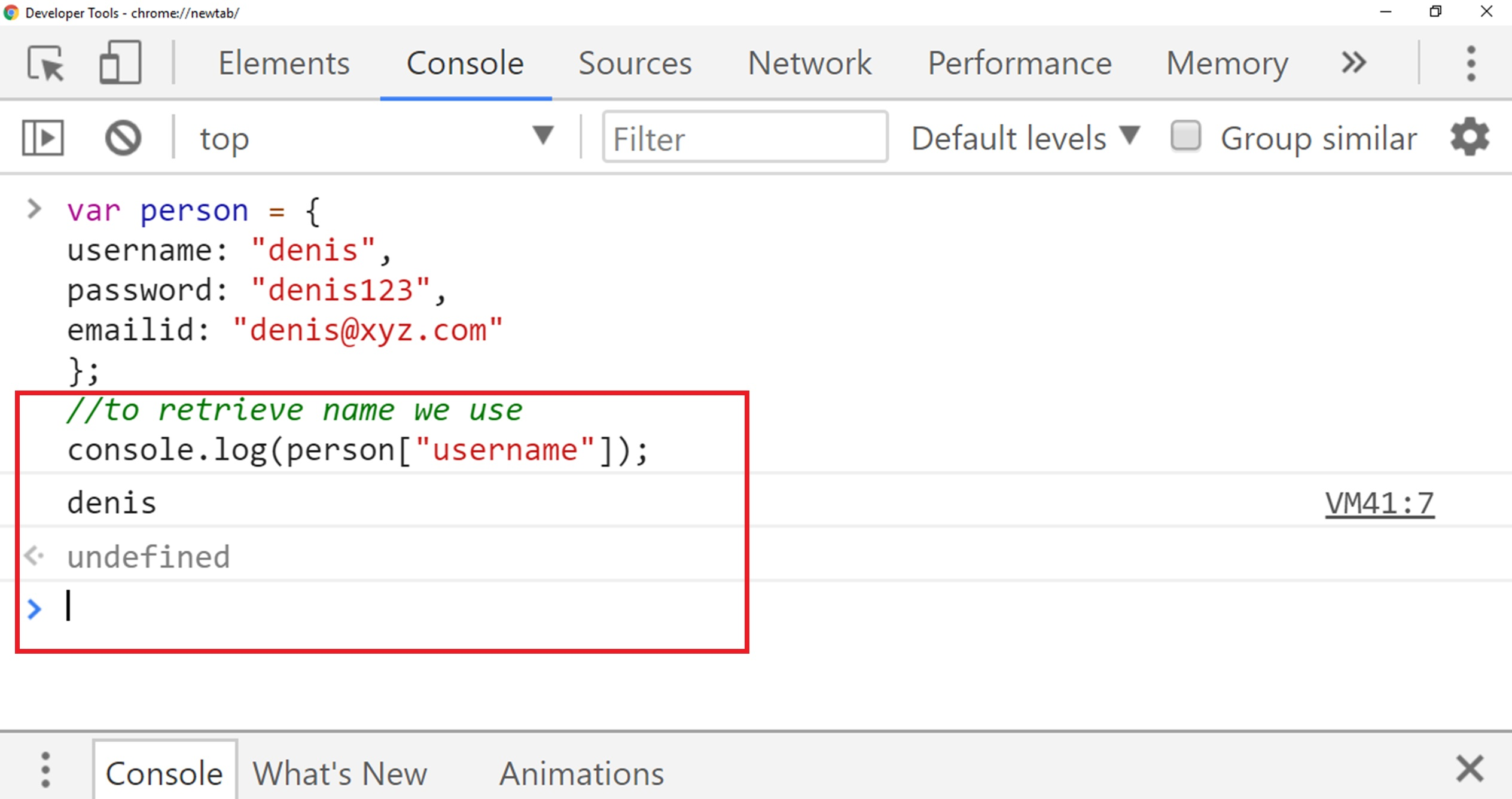So that’s very similar to arrays and the only difference is that we passing string that is username instead of index number.
The other way to retrieve data from the object is a little bit shorter and simple, so to retrieve data we use object name followed by a dot.
And then key value like the username.
Here is the code below:

``````var person = {
emailid: "denis@xyz.com"
};
``````

Here is the output below: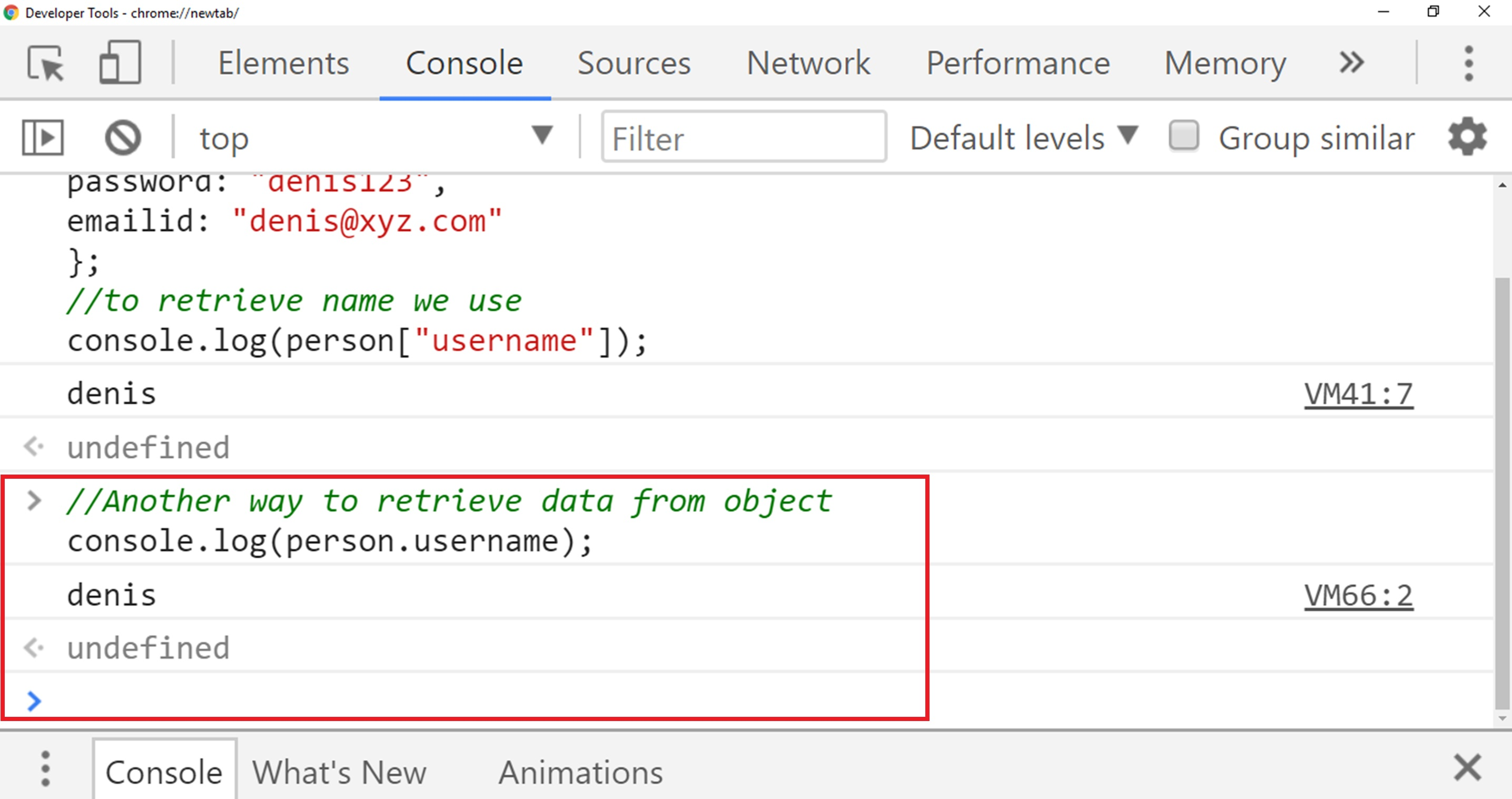## Updating Data of an object

The next thing we want to do is updating our object data so if I want to update the password, so it is very similar to arrays where we can access the data and then reassign it with an equal sign.
In our case, we want to update our password key which has value “denis123” to “xyz123” so to do that we use person.password and then reassign password to “xyz123”.
so here is the code below:

``````var person = {
emailid: "denis@xyz.com"
};
//Now password changed from denis123 to xyz123
``````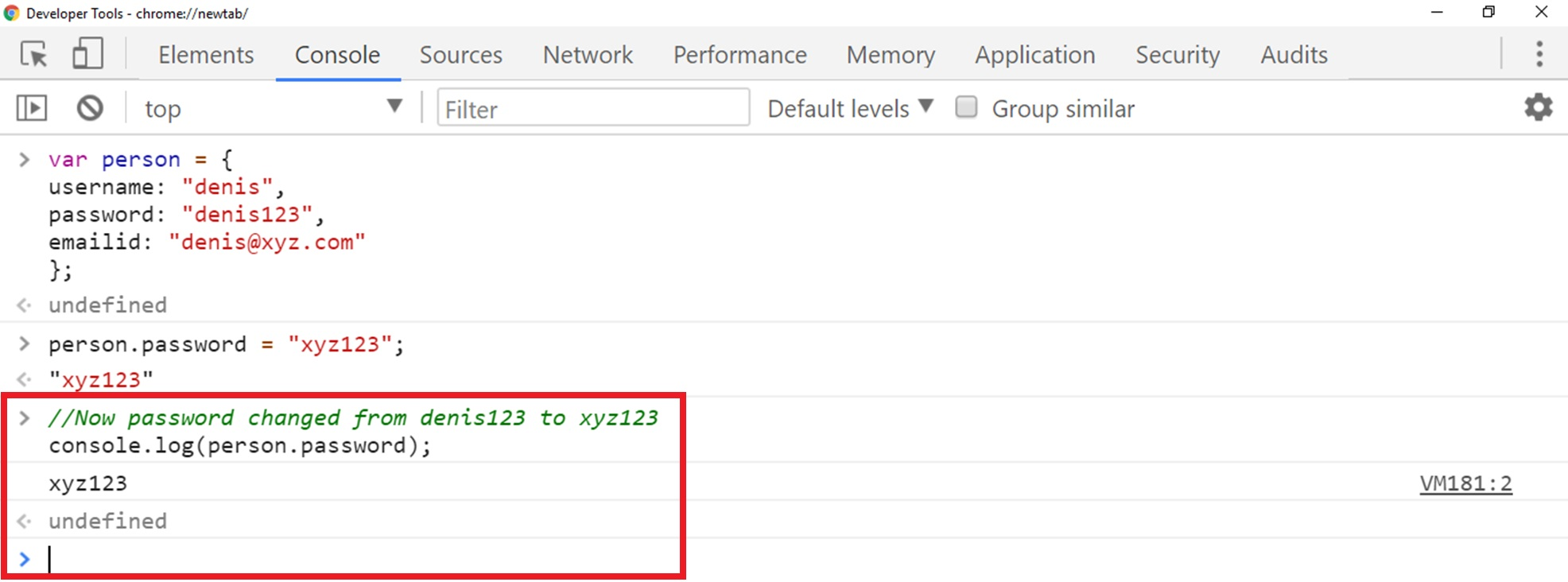There is another way that you can change the value.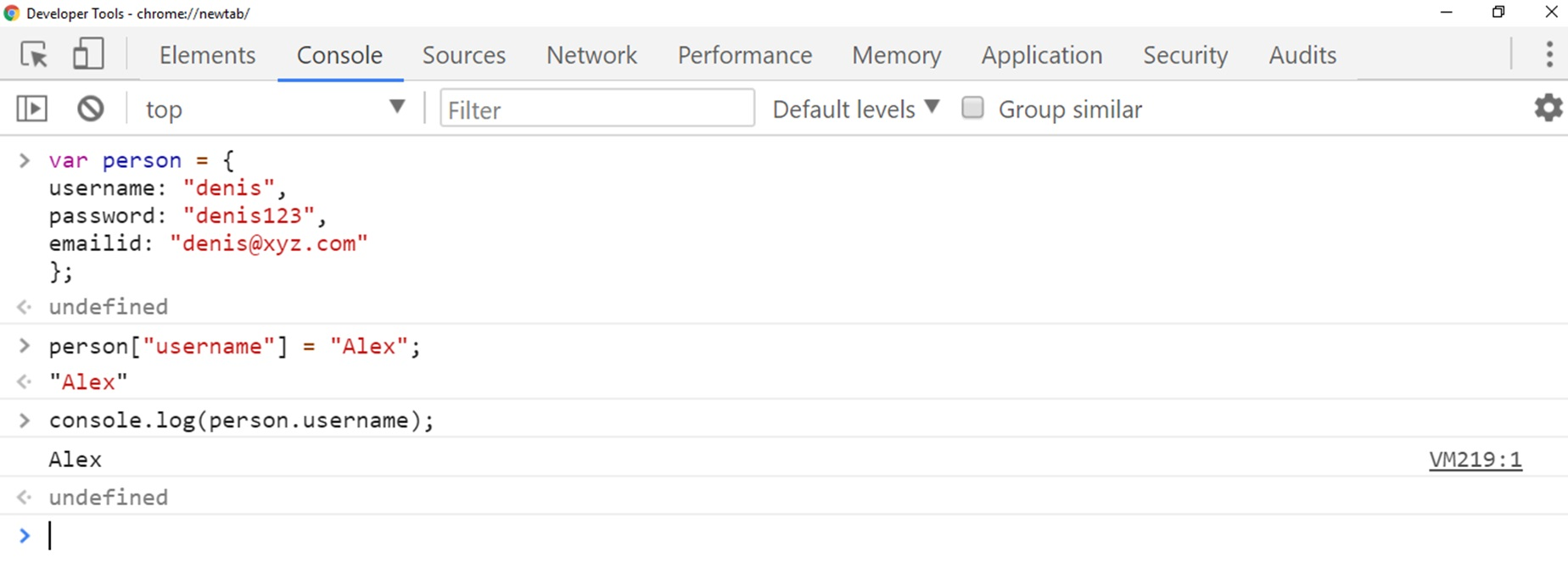## Conclusion

In this lesson, we have discussed Objects in JavaScript which is also a part of data structure and we have also discussed what objects are and how we can use objects in our program and learned how we can change the value of an object.